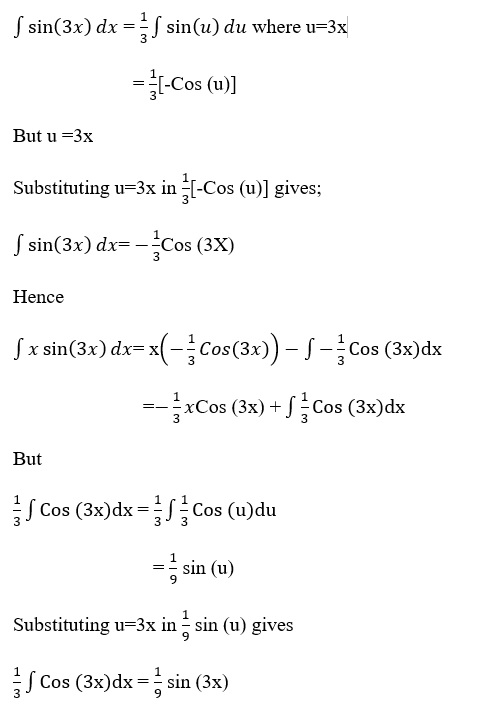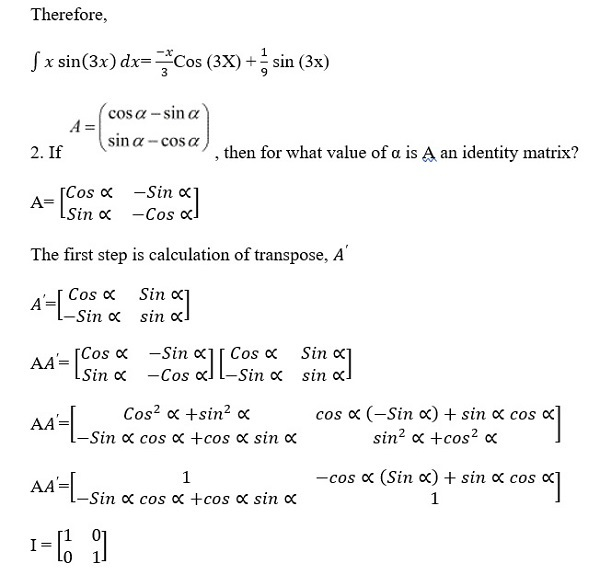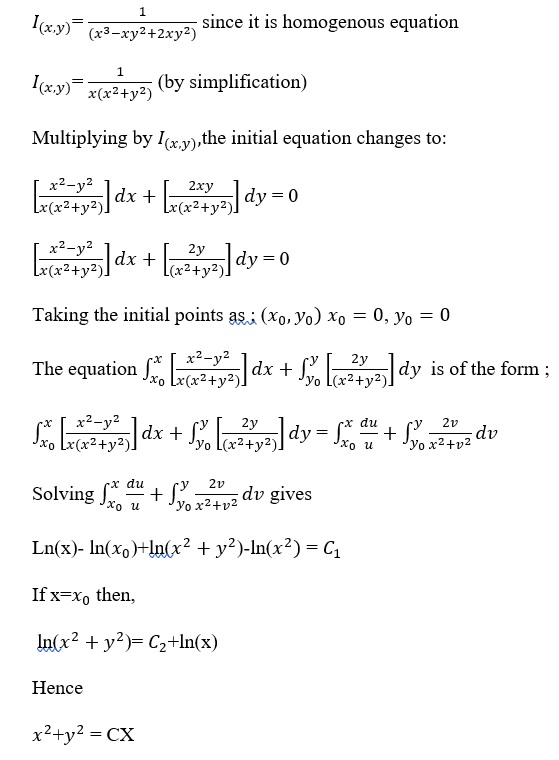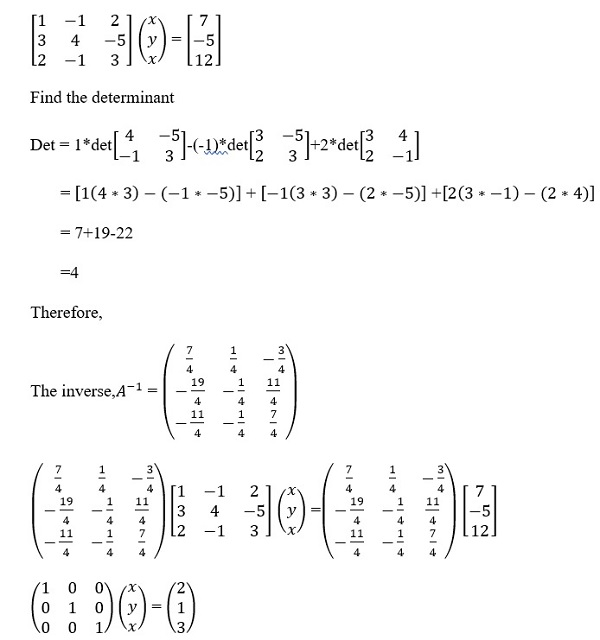# Math subjective test 2

Provide solution to the following questions:

1. Evaluate the following: ∫xsin3xdx

This question requires integration by parts

Let u=x and dv/dx =sin (3x)

du=x dx=1

But1. The line y = mx + 1 is a tangent to the curve y2= 4x .Find the value of m.

Substitute y=mx+1 in =4x

= 4x

Expanding = 4x

+1+2mx-4x=0

+x(2m-4)+1=0

The tangent touches the curve at one point hence the roots are equal. Therefore, discriminant =0

-4((1) = 0

+16-16m-=0

16-16m=0

16=16m

M=1

1. Solve the following differentialequation:(x2y2) dx + 2 xy dy = 0.

Te integrating factor of the equation is1. Solve system of linear equations, using matrix method.

xy +2z =7
3x +4y −5z =−5
2x y + 3z = 12Hence

X=2

Y=1

Z= 3

Reference

Chirgwin, B., & Plumpton, C. (2016). A Assignment of mathematics for engineers and scientists. Elkins Park: Pergamon Press/Elsevier Science.